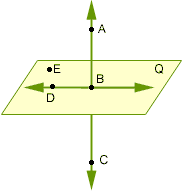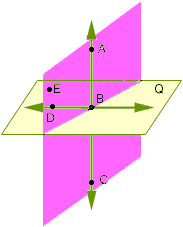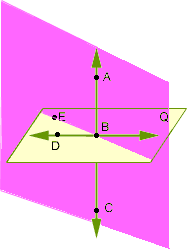Grade: 9 Age: 14 Who is asking: FatherHow many planes contain each line and point? BD and point A  AB and point C  BE and point C  BD and point E  Hi Harold, There are three of important facts here that show you how to solve this problem. First, if you have three distinct points in 3-space then there is a plane that contains these three points. Second, if the three points lie on a line then there are in fact infinitely many planes that contain the three points. For example if the three points are A, B and C in your diagram then there are infinitely many planes that contain the points. I have illustrated two such planes in pink in the diagrams below.The final point is that if the three points do not lie on a line then there is exactly one plane that contains the points. For example if the points are A, B and E then, in the diagram on the right above, the plane coloured pink is the only plane that contains the three points. I hope this helps, Harley Go to Math Central